# Steering Behaviors: Seek

This essay offers a concise explanation with regards to steering behaviors, particularly seek.

## Seek

The goal of Seek Steering Behavior is to steer the character towards its target goal. This is especially important for autonomous characters in the game world who may need to pursue the character during a chase or go to the store. Seek behavior brings them to life making them intelligent. This behavior is more commonly applied in instances where you have an autonomous character following the main character or they are leading the main character to a destination.

## In a Nutshell

The image above shows the mathematical translation of the seek behavior. The velocity vector represents the direction and motion to the target. It is made up of x and y components that have a hypotenuse which forms a right angle triangle. This information gives us the tools we need to calculate the velocity vector(hypotenuse) the object needs to reach its target.

We decompose the velocity vector or hypotenuse which gives us the x and y components values. These are used to add to the NPC’s current position updating and advancing the position in time.

position = position + velocity

position(x,y) = position(x,y) + velocity(v_x,v_y)

## Important to Understand

When dealing with vector math, it’s best to think of everything on a cartesian plane (x,y) for 2D or (x, y, z) plane for 3D. Remember the x and y coordinates are arbitrary. They represent any values we want them to be as long as they are related to each other with the independent variable going along the x-axis and the dependent variable on the y-axis.

In the case of distance traveled and time spent traveling. The time changes as distance increases or decreases, this means that time depends on distance. The distance does not increase or decrease with time, which means that it is not affected by time. Therefore in the world of game development the x-axis will represent the position and the y-axis usually the time or any other dependent variable.

## 3D Math

In 3D Math, we decompose or simplify the world by imposing a cartesian coordination with x, y and z axis on it, the origin can be anywhere we wish. The z-axis represents the space element or depth of the world, this tells us that the world has volume or 3 dimension.

The x and y axis gives geometric data about the world. Geometry is the branch of mathematics concerned with spatial relationships of relations of object in a space, which in simple terms can be imagined as a plane with objects placed across the surface. These objects have position or location, depth and range if they move across the plane. We use these attributes to gather information about the plane “world” and the objects that inhabit it and do maths.

## Movement

Let’s say the plane has a moving object such as a car that happily exists in the plane world unaware of our big brother tendencies.

The car is parked at home and this is a point on the plane, because remember everything that exists on a cartesian coordinate has a position associated with it on the x-axis.

Let’s say the car is at position x = 10. Units don’t matter in this case, but they do when you are building a space shuttle or being extremely specific.

The value of the y-axis will depend on what we want to know about the car. If we wanted to know the car’s location, the y-axis would represent the orientation pair value. The y-axis would represent the North-South orientation and the x-axis would represent the West-East orientation. A cartesian coordinate becomes whatever you need it to become.

In this case we want to know more about time taken to travel to the grocery store from home. The y-axis becomes a function of time or represents time.

This allows us to gather the information (x, y) coordinates and find the velocity it takes for the car to go from home to the grocery store.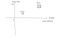A 2d plane has been superimposed on the world like tracing paper and now we have the location of home and the store on the 2D plane.

## What?!>?

We can draw a line to represent the distance from the point home to point grocery store. In this case, the grocery store is 8–6 = 2 miles away from home.

When we draw a vertical line, this line will run parallel to the y-axis which in math means it is related to the y-axis and gives us information about the y-axis. In this case it gives us information about the change in time from the position of point home to the position of point store.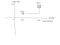This vertical line could have been drawn anywhere on the plane if we didn’t need the hypotenuse and it was only used to represent the time .As long as it started on the same horizontal line as home and ended on the same horizontal line as store.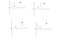If this was read-only the line would be used as a measure of the change in time. But we want more from our data, we want to find how fast the car traveled in that amount of time to get to the store. Which is why we connect the vertical line to the start point and connect it’s end to the destination, which forms a triangle.

When we get the direction from home to the store and draw a direct path from home to the store we connect the change in the y-axis from the home point to the store, we form a right-angled triangle, which brings us into the world of trigonometry which allows us access to direction.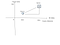This is correct

We can draw or join the end time to the destination to the start time at the starting point forming a right-angled triangle which looks familiar. Hello Pythagoras, gaining more abilities.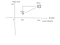This is correct too. As long as you maintain the integrity of the right angled triangle with the y-axis drawn vertically, the x-axis parallel horizontal. The hypotenuse becomes the line connecting the end of the time taken vertex to the store or home vertex. Resulting in a connection.

Now that we have a change in x and a change in y forming a right angled triangle with a hypotenuse we can access the pythagorean theorem because we have all the data needed to calculate it.

Math is about data collection. Figure out what data you have and then find a formula that uses the data, which will give you access to more information that will get your result or closer to it.

The hypotenuse of a right-angled triangle can be used in many mathematical questions. For our needs we will use it to represent velocity. This is because velocity is a mathematical idea forming an arrow that is made up of magnitude and direction, which are made up by run/rise which result in a hypotenuse via pythagorus.

The hypotenuse can represent velocity which is a vector has magnitude and direction. Remember velocity is the change in displacement(position) / change in time and the right angled triangle shows us that a change in run or x-axis values and a change in rise or y-axis values can be used to find the hypotenuse.

Apply this to the right angled triangle results in access to the velocity components V(x) and V(y).

Velocity is a vector that exists in space, which means that it has [x, y, z], we will ignore the z for now.

V(x) is associated with the x-axis and V(y) the y-axis giving us the horizontal and vertical directions. Adding these two together using pythagorus gives us the direction or hypotenuse which is also the velocity.

Easy.

## Calculus, we are here

In order to calculate the character’s new position seek uses the Euler Integration which simply calculates the next position based on the data from the current position and adds these values to find the sigma or changing sum of the variables.

## How It’s Done

The velocity vector is sliced into small components via normalizing the vector. This results in a unit vector or vector with a length of 1 or less. We take this vector and convert it to a hypotenuse, which allows us to access the x and y components which are added to the current position and used to find the final position.

Remember, this is possible because velocity is made up of (v(x), v(y)) components which are added to position (x, y), advancing us to the next position.

position = position + velocity

The size of the vector will determine how fast the character moves and the direction will control where the character is headed. Normalizing the velocity truncates it to ensure it remains and moves realistic in steering behaviors.

The whole point of understanding this math, is to understand how things change infinitesimally or microscopically.

This is Calculus.

The study of small changes derivatives and the study of adding up these small changes to get a picture of the whole is called integration.

## Calculus and Basic Maths

When we learn basic math, we are taught to see the whole rather than the details. Calculus is about the small details or what it took to get there.

For example. A car travels a distance of 1200meters over a period of 400seconds. How fast was it going?

speed = distance / time = 1200/ 400 = 3m/s

This tells us that the car covered 3 meters every 1 second using basic math we see the whole picture without details.

## Details

Calculus allows us to slow things down so we can observe them better. A car traveling 3m/s is going very fast, the normal human eye would miss it, but if we say the current position is 0, meaning the car just started.

distance(position) = 0, time(0) which gives us a coordinate of (0,0) the origin.

velocity(3, 1) because 3m runs along the x-axis and 1second represents the y-axis.

we can now use the Euler’s Integration(math is about gathering data and figuring out which formulae use the data)

position = position + velocity — will give us the new position allowing us in our case to create realistic characters and animations otherwise everything would move too fast for the players.

new_position = (0, 0) + (3, 1) = (3, 1)

Doing this calculation over a period of 400s

new_position = (3, 1) + (3, 1) = (6, 2)

new_position = (6, 2) + (3, 1) = (9, 3)

…until time = 400s, meaning the y-axis value gets to 400 will give us second by second positions of the car the infinitesimal changes.

# Adding Forces

So far the character will move straight line and turn abruptly which is not very convincing. To make it so we apply forces.

Forces are the push or pull on an object resulting in steering behaviors the applied forces will take the character in the direction of the thing it seeks. If your player character is being chased, your NPCs will run after them and should be able to modify their direction when using Euler’s integration which means the values associated with velocity change and must now be modified to take the NPC on a new path towards the player, otherwise they will keep going straight.

## Giving chase

To accomplish this, we take the current position and use it to determine the direct path to the character will move to the target, this is the desired velocity.

Since the character already has a current velocity which is leading them to the past player position, we use steering forces to steer the character from this path to the new target by first finding the desired_hypotenuse or velocity because the path between the character’s current position and the target has changed.

We use the following formula.

desired_velocity = normalize(target_position — position) * max_velocity

We are simply finding the new hypotenuse which is the velocity.

Normalizing is a mathematical formula applied to vectors. It allows us to take a vector of any length and keep it pointing in it’s authentic direction but modifying it’s length to 1. Normalizing gives us a unit vector which keeps our magnitude within realistic margins.

Basically, we normalize velocity to keep the speed realistic while maintaining direction, otherwise it will move funny.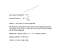Slow your roll

This means that the position will be increased by 0.95 and 0.32 respectively while maintaining the original direction.

## Steering Force

The steering force gives us the amount or numbers to add to the current position so that the character’s direction can change to reach the target’s new position.

This is calculated by subtracting the desired_velocity vector from the current_velocity vector.

We can now use the vector subtraction advantage.

Remember that subtracting two vectors is like pulling them apart which results in a new vector in between them. Because of calculus we can have the small changes of this vector and add these to the current position to create the new direction.

steering = desired_velocity — velocity

The new vector called steering is applied in increments using Euler’s integration and this results in a new path.

## To Be Continued…

Game Developer. Computer Scientist. My fur baby calls me Genie-Momma. Yoga Teacher. Fun is Non-Fiction/ Fiction. Ice Cream Connoisseur. Lover of living best.

## More from Mbozu Grace Lweendo

Game Developer. Computer Scientist. My fur baby calls me Genie-Momma. Yoga Teacher. Fun is Non-Fiction/ Fiction. Ice Cream Connoisseur. Lover of living best.